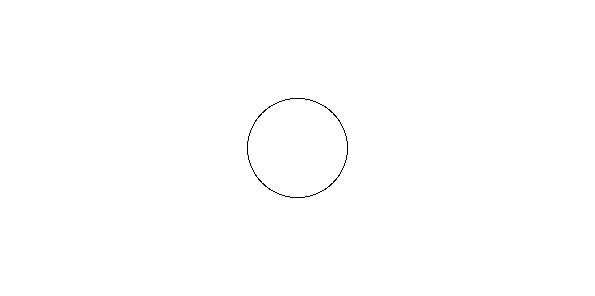# Plot a circle using centre point and radius in MATLAB

The aim is to plot a circle using center point and radius in MATLAB without using inbuilt functions for plotting. A black and white image can be represented as a 2 order matrix. The first order is for the rows and the second order is for the columns, the pixel value will determine the color of the pixel based on the grayscale color format.

Approach :

• We are given with a point and radius. Let the coordinates of the centre point be (x1, y1) and radius be R.
• We find the distance of centre point to every pixel(i, j)th.
`dist = sqrt((j-c)^2+(i-r)^2);`
• Now if dist=R i.e radius we make that pixel black.

Below is the implementation:

 `% MATLAB code to plot circle using centre and radius. ` ` `  `% create a white image of size 300X600 ` `I=zeros(300, 600)+1; ` ` `  `% Radius of circle ` `R=50; ` ` `  `% coordinates of centre point ` `c=300; ` `r=150; ` ` `  `% accessing every pixel ` `for` `i=1:size(I, 1) ` `    ``for` `j=1:size(I, 2) ` `        ``dist=round(sqrt((j-c)^2+(i-r)^2)); ` `        ``if` `dist==R ` `            ``I(i, j)=0; ` `        ``end` `    ``end` `end` ` `  `% display the image ` `figure, imshow(I); `

Output :My Personal Notes arrow_drop_upCheck out this Author's contributed articles.

If you like GeeksforGeeks and would like to contribute, you can also write an article using contribute.geeksforgeeks.org or mail your article to contribute@geeksforgeeks.org. See your article appearing on the GeeksforGeeks main page and help other Geeks.

Please Improve this article if you find anything incorrect by clicking on the "Improve Article" button below.

Article Tags :

Be the First to upvote.

Please write to us at contribute@geeksforgeeks.org to report any issue with the above content.